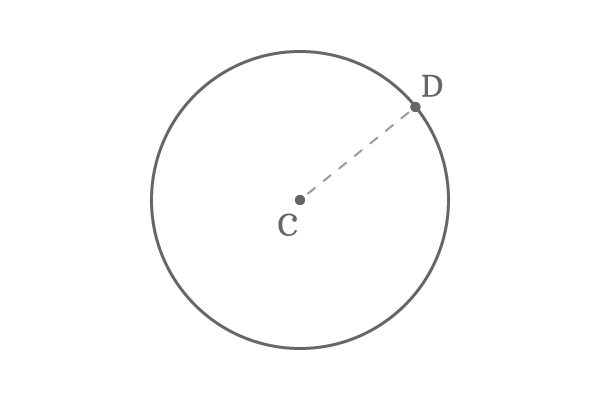The distance form centre to any point on the circumference of a circle is called radius of the circle.

## IntroductionEvery point on the circumference of the circle maintains a constant distance from centre of the circle. The constant distance between them is called as radius. It is popularly denoted by letter $r$ in geometry.

Actually, the distance between two points is same as the length of the line segment which joins two points. Hence, the radius of a circle is geometrically denoted by a line segment which joins centre and any point on the circumference.

### Example

$C$ is a centre of a circle and $P$ is any point on the same circle.According to definition of radius of a circle, the distance from centre $C$ to any point $P$ on the circumference is known as radius of the circle. If they are joined by a line, then the length of line segment is same as the distance between them.

It means, the radius of a circle is equal to the length of the line segment $\overline{CP}$. Hence, the radius in a circle is denoted by a line segment geometrically.

$\therefore \,\,\,\,\,\, CP \,=\, r$

Latest Math Topics
Jun 26, 2023
Jun 23, 2023

###### Math Questions

The math problems with solutions to learn how to solve a problem.

Learn solutions

Practice now

###### Math Videos

The math videos tutorials with visual graphics to learn every concept.

Watch now

###### Subscribe us

Get the latest math updates from the Math Doubts by subscribing us.